Publication date: 11/10/2021

## Calculations for Outlier Analysis

The calculations in the Outlier Analysis report use the following notation:

n = number of observations

A = number of principal components

Xci = the standardized data for the ith observation

### T2 Statistic

The T2 statistic for the ith observation is calculated as follows: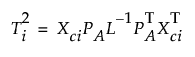where PA is a matrix containing the first A eigenvectors and L is a diagonal matrix containing the first A eigenvalues.

The median and UCL for the T2 plot are calculated as follows: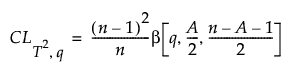where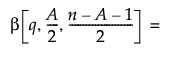the qth quantile of the Beta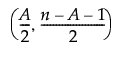distribution.

To calculate the median, use q = 0.5. To calculate the UCL, use q = (1 - α).

### Contribution Statistic

The vector of T2 contribution statistics for the ith observation is calculated as follows: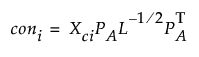Note: The sum of the squared contributions for an individual is equal to Ti2 for that individual.